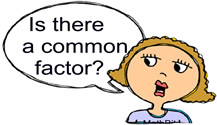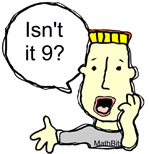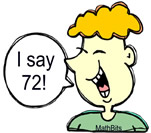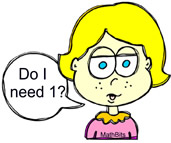Common Factors Terms of Use    Contact Person: Donna RobertsDirections: Answer these questions pertaining to common factors. The cartoon people may, or may not, be helpful!!

1.
What is the greatest common factor (GCF) of
30 and 45?

Choose:
 5 9 15 182.
What is the greatest common factor (GCF) of 7 and 15?

Choose:
 1 3 5 73.
What is the greatest common factor (GCF) for 9, 21, and 63?

Choose:
 3 7 9 124.
What is the least common multiple (LCM) for 2, 4 and 9?

Choose:
 8 18 36 725.
Two numbers have a greatest common factor of 8, and a least common multiple of 48. If one of the numbers is 16, what is the other number?

Choose:
 3 18 24 366.
What are the common factors of 15 and 45?

Choose:
 5, 15 1, 3, 5, 15 3, 5, 15 1, 3, 5, 9, 157.
Use the distributive property to find the greatest common factor in the expression 6x + 18.

Choose:
 2 3 6 98.
Factor using the distributive property to find the greatest common factor: 0.33m + 0.99

Choose:
 0.33(m + 3) 0.3(0.11m - 0.33) 0.33(m + 0.99) 0.3(1.1m - 3.3)9.
What are the factors of 121?

Choose:
 1, 121 1, 3, 121 1, 3, 7, 121 1, 11, 12110. Which of the following expressions is (are) NOT equivalent to -6x + 12y? (Check all that apply, and hit SUBMIT!) -6(x - 2y) -3(2x - 4y) 2(-3x + 6y) ½(-12x + 24y) -6(x + 2y)